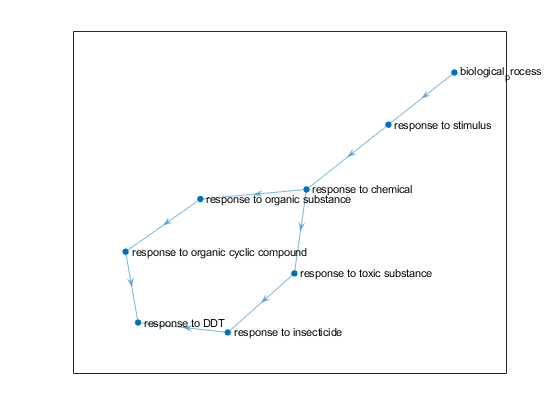# getmatrix

Convert `geneont` object into relationship matrix

## Syntax

``[Matrix] = getmatrix(GeneontObj)``
``[Matrix,ID] = getmatrix(GeneontObj)``
``````[Matrix,ID,Relationship] = getmatrix(GeneontObj)``````

## Description

example

````[Matrix] = getmatrix(GeneontObj)` converts a `geneont` object into a matrix of relationship values between nodes (row and column indices).```

example

````[Matrix,ID] = getmatrix(GeneontObj)` also returns a column vector listing Gene Ontology IDs that correspond to the rows and columns of `Matrix`.```

example

``````[Matrix,ID,Relationship] = getmatrix(GeneontObj)``` also returns a cell array of character vectors defining the types of relationships.```

## Examples

collapse all

Download the current version of the Gene Ontology database from the Web into a `geneont` object.

`GO = geneont('Live',true)`
```Gene Ontology object with 47266 Terms. ```

Retrieve the ancestors of the Gene Ontology term with an identifier of 46680.

`ancestors = getancestors(GO,46680)`
```ancestors = 8×1 8150 9636 10033 14070 17085 42221 46680 50896 ```

Create a subordinate Gene Ontology.

`GeneontObj = GO(ancestors)`
```Gene Ontology object with 8 Terms. ```

View the relationships in `GeneontObj`.

`[Matrix,ID,Relationship] = getmatrix(GeneontObj)`
```Matrix = (6,2) 1 (6,3) 1 (3,4) 1 (2,5) 1 (8,6) 1 (4,7) 1 (5,7) 1 (1,8) 1 ```
```ID = 8×1 8150 9636 10033 14070 17085 42221 46680 50896 ```
```Relationship = 1×6 cell {'is_a'} {'negatively_regulates'} {'part_of'} {'positively_regulates'} {'regulates'} {'term_tracker_item'} ```

Plot the relationships.

```BG = digraph(Matrix,get(GeneontObj.terms,"name")); plot(BG,Layout="force")```## Input Arguments

collapse all

Gene ontology object, specified as the output of the `geneont` command.

Example: `geneont('Live',true)`

## Output Arguments

collapse all

Relationship values between nodes (rows and columns) of `GeneontObj`, returned as a sparse matrix with the following entries:

• `0` — No relationship

• `1` — “is_a” relationship

• `2` — “part_of” relationship

Gene ontology IDs, returned as a nonnegative integer column vector. The entries in `ID` correspond to the entries in `Matrix`.

Types of relationships, returned as a cell array of character vectors.

## Version History

Introduced before R2006a